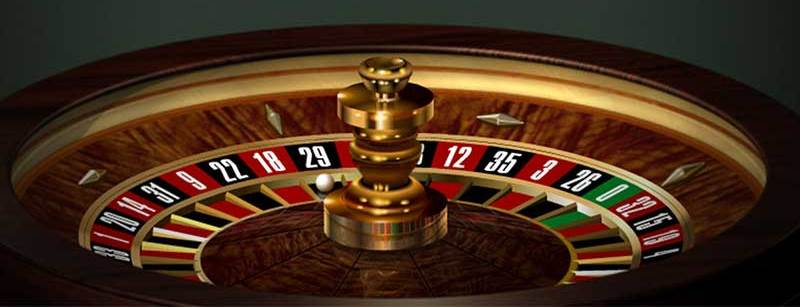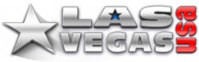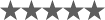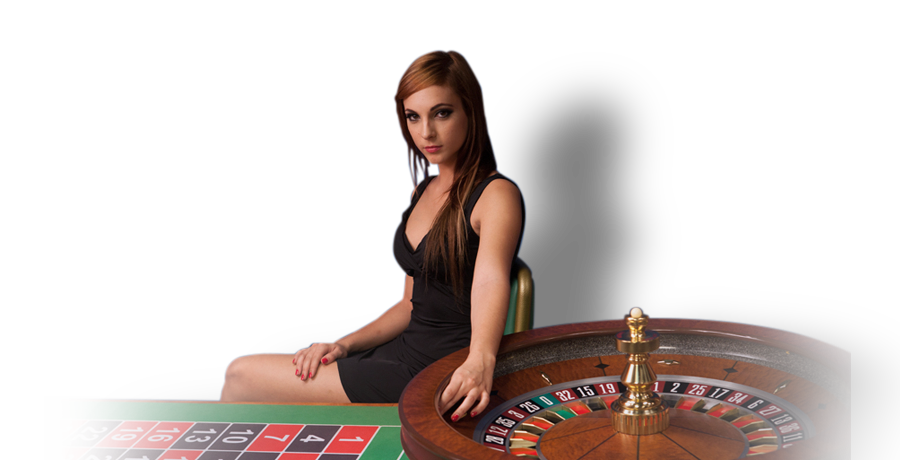# Roulette Pattern Betting

While it is true that the roulette wheel has no memory, can't think or feel, and has no sense of time or space, table results often produce patterns that appear to be consistent or predictable.

With even-money bets such as red or black or odd for roulette and the banker and player bets for baccarat, innumerable patterns routinely appear. Can we make any money trying to use these patterns for predictive purposes or are we just deluding ourselves?### Our Favorites Casino sites for 2023### Bonus up to \$3,000

The best daily bonuses on the internet

Our score:(4.3 / 5)### 150% up to \$10,000

Welcome Bonus

Our score:(4.2 / 5)### 250% Bonus up to \$1,000

Welcome Bonus

Our score:(4.2 / 5)### 400% up to \$10,000

Bonus Code: 400BONUS

Our score:(4.2 / 5)### 400% up to \$10,000

Bonus Code: 400BONUS

Our score:(4.2 / 5)

## Patterns and Probabilities

Many gambling systems are based on observing the outcome of casino wagers and then either betting with the trend or betting for the trend to end.

Assume that you and I are sitting at a roulette table and we observe that the wheel has landed on a red number for the last three spins. If we are of the school of thought that this signals that another red number is due, we will probably bet for red to repeat.

However, we may believe that any event occurring in a casino game is of limited duration and decide to wager that a black number shows, ending the streak of red numbers.

Neither of these systems has any statistical validity, as the occurrences of red or black numbers on a roulette wheel are what statisticians call independent events.

In general, two or more events are said to be independent of each other if the occurrence of one in no way affects the probability of the occurrence of any of the others.To give another illustration, let's determine the probability of drawing two kings in succession from a deck of 52 ordinary playing cards, without the first card being replaced before the second is drawn. Since there are four kings, the probability of getting a king on the first draw is 4/52. Given that the first card drawn is a king, the probability of getting a king on the second draw is 3/51, reflecting that we only have three kings left out of 51 cards. In this case, the probability of drawing the second king is dependent on the outcome of the first draw. We could calculate the probability of getting two kings in a row as 4/52 x 3/51 = 1/221.

If we had replaced the first card before the second was drawn, the probability of getting a king on the second draw would have been 4/52 (the same as getting a king on the first draw). We could then compute the probability of getting two kings in a row under these circumstances as 4/52 x 4/52 = 1/169.

Since the probability of getting a king on the second draw is now 4/52 regardless of what happened on the first draw, these draws are independent. Generally speaking, two or more events are independent if the occurrence of one in no way affects the probability of the occurrence of any of the others.

If two events are independent, the probability that they will both occur is the product of their respective probabilities. With a balanced coin, the probability of getting heads is 1/2 and the probability of getting two heads in two flips is 1/2 x 1/2 = 1/4. The probability of getting four heads in a row is 1/2 x 1/2 x 1/2 x 1/2 = 1/16.

Returning to our example of three red numbers in a row, if we assume that the probability of spinning a red or black number is 1/2, then the probability of the next spin being another red is 1/2. Likewise, the probability of the next spin being a black number is also 1/2. Because the result of each spin is independent of each other spin, we find that the previous spins have no effect on the outcome of the next spin.

If we examine this problem from a different angle and ask what the probability is of getting four red numbers in a row, we find that it is 1/16, the same probability of flipping four heads in a row with a coin. If we ask what the probability is of spinning at least one black number in four spins, we find that probability is 15/16.

With the casino games of craps, roulette, and baccarat, we are dealing with independent events, where the outcome of a previous decision does not affect the following decision. With blackjack, we are dealing with dependent events, for as we saw when drawing kings out of a deck, if we don't replace the drawn cards after each draw, the probability of the next draw will change.

This is the reason that blackjack is considered a game of skill while the other casino games are considered games of chance. With skill, we can alter our strategy as the probabilities change in a blackjack deal, while with the games of chance, we should probably keep the same strategy throughout a game. (Technically, baccarat is also a game of skill as the probabilities change as cards are dealt, but because of the mechanics used for playing the game, it can for all practical purposes be treated as a game of chance, which we have done).